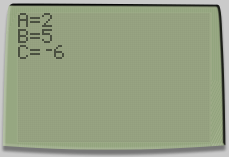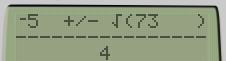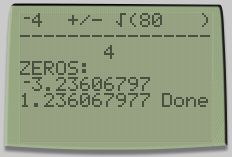One of the most useful programs you can have in your TI calculator is the Quadratic Formula.

During this tutorial, I will teach you how you can do your own program to calculate the solutions of any equations of the following type:

$$ax^2+bx+c=0$$

First, we need to get $$a$$, $$b$$ and $$c$$ values from user. To achieve this we will use some Input instructions preceded by a ClrHome one, that tells the processor to clear the screen.

ClrHome
Input "A=",A
Input "B=",B
Input "C=",C

These “inputs” retrieve the values from the user and store them in the variables A,B and C.Then we will clear the screen once more and add some Disp “” instructions that will be discussed later.

ClrHome
Disp ""
Disp ""
Disp ""
Disp ""
Disp ""

Now it’s time to do the intermediate step:

$$\dfrac { -b\pm \sqrt { { b }^{ 2 }-4ac } }{ 2a }$$

We will use Output instructions to display text and variable values to a specific place on the screen:

Output(1,1,­B)
Output(1,5,"+/-")
Output(1,9,"√(")
Output(1,11,B²-4AC)
Output(1,16,")")
Output(2,1,"----------------")
Output(3,8,2A)And finally we check if the equation has solutions by checking if the following inequation is true:

$${ b }^{ 2 }-4ac\ge 0$$

If it is true, then we can print the solutions. However, if it has no solutions, we should print an error message like “NO ZEROS FOUND”. The Then and End instructions mark the beggining and the end of a block, which means that if the condition is true, all the instructions before the Else will be executed and if it is false it will only execute the instruction between Else and End.

Output(4,1,"ZEROS:")
If (B²-4AC)≥0
Then
Output(5,1,(­B-√(B²-4AC))/(2A)
Output(6,1,(­B+√(B²-4AC))/(2A)
Else
Output(5,1,"NO ZEROS FOUND"
End
Output(5,12," "
Output(6,12," "

The last Output instructions limit the solution digits presented.To conclude, we should note that the previously used Disp “” instructions just tell the processor to print Done in a specific place in the screen.

Here you have the full code:

ClrHome
Input "A=",A
Input "B=",B
Input "C=",C
ClrHome
Disp ""
Disp ""
Disp ""
Disp ""
Disp ""
Output(1,1,­B)
Output(1,5,"+/-")
Output(1,9,"√(")
Output(1,11,B²-4AC)
Output(1,16,")")
Output(2,1,"----------------")
Output(3,8,2A)
Output(4,1,"ZEROS:")
If (B²-4AC)≥0
Then
Output(5,1,(­B-√(B²-4AC))/(2A)
Output(6,1,(­B+√(B²-4AC))/(2A)
Else
Output(5,1,"NO ZEROS FOUND"
End
Output(5,12," "
Output(6,12," "